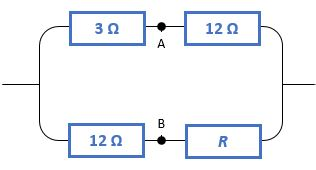# Path of Less ResistanceWhen this circuit is connected to a certain voltage source, a current of $21 \text{ mA}$ runs through it.

But when points $A$ and $B$ are connect by a wire, the current in the circuit increases to $25 \text{ mA}$.

Determine the value of resistance $R$ in ohms.

×